# KSEEB Solutions for Class 8 Maths Chapter 4 Factorisation Additional Questions

Students can Download Maths Chapter 4 Factorisation Additional Questions and Answers, Notes Pdf, KSEEB Solutions for Class 8 Maths helps you to revise the complete Karnataka State Board Syllabus and score more marks in your examinations.

## Karnataka State Syllabus Class 8 Maths Chapter 4 Factorisation Additional Questions

Question 1.
(a) 4a + 12b is equal to
A. 4a
B. 12b
C. 4(a + 3b)
D. 3a
(C) 4 (a + 3b)(b) The product of two numbers is positive and their sum is negative only when
A. both are positive
B. both are negative
C. one positive other negative
D. one of them is equal to zero
(B) both are negative

(c) Factorising x2 + 6x + 8 we get
A. (x + 1) (x + 8)
B. (x + 6) (x + 2)
C. (x + 10) (x – 2)
D. (x + 4) (x + 2)
(D) (x + 4) (x + 2)

(d) The denominator of an algebraic fraction should not be
A. 1
B. 0
C. 4
D. 7
(B) 0

(e) If the sum of two integers is – 2 and their proiduct is – 24, the numbers are
A. 6 and 4
B. – 6 and 4
C. – 6 and -4
D. 6 and – 4
(B) – 6 and 4

(f) The difference (0.7)2 – (0.3)2 simplifies to
A. 0.4
B. 0.04
C. 0.49
D. 0.56
(A) 0.4Question 2.
Factorise the following
(i) x2 + 6x + 9
x2 + 6x + 9
= x2 + 2.x.3 + 32
= (x + 3)2

(ii) 1 – 8x + 16x2
1 – 8x + 16x2
= 12 – 2.1.4x + (4x)2
= (1 – 4x)2

(iii) 4x2 – 81y2
4x2 – 81 y2
= (2x)2 – ( 9y)2
= (2x + 9y) (2x – 9y)

(iv) 4a2 + 4ab + b2
4a2 + 4ab + b2
= (2a)2 +2.2a.b + b2
= (2a + b)2

(v) a2b2 + c2d2 – a2c2 – b2d2
a2b2 + c2d2 – a2c2 – b2d2
= a2b2 – a2c2 + c2d2 – b2d2
= a2 (b2 – c2) – d2 (b2 – c2)
= (b2 – c2) (a2 – d2)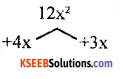Question 3.
Factorise the following
(i) x2 + 7x + 12
x2 + 7x + 12 12xz
x2 + 4x + 3x + 12

x (x + 4) + 3 (x + 4)
(x + 4) (x + 3)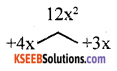(ii) x2 + x – 12
x2 + 4x – 3x – 12
x(x + 4) -3 (x + 4)
(x + 4) (x – 3)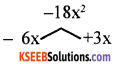(iii) x2 – 3x – 18
x2 – 6x + 3x – 18
x(x – 6) +3 (x -6)
(x – 6) (x + 3)(iv) x2 + 4x – 21
x2 + 7x – 3x – 12
x(x + 7) -3 (x + 7)
(x + 7) (x – 3)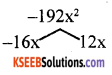(v) x2 – 4x – 192
x2 – 16x + 12x – 192
x(x – 16) +12 (x – 16)
(x -16) (x +12)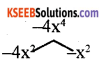(vi) x4 – 5x2 + 4
x4 – 4x2 – x2 + 4
x2(x2 – 4) -1 (x2 – 4)
(x2 – 4) (x2 – 1) = (x + 2) (x – 2)
(x + 1) (x – 1)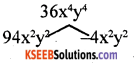(vii) x4 – 13x2y2 + 36y4
x4 -9x2y2 – 4x2y2 +36y4
x2(x2 – 9y2) -4y2
(x2 – 9y2)
(x2 – 9y2) (x2 – 4y2)
= [x2 – (3y)2] [x2 – (2y)2]
= (x + 3y) (x – 3y) (x + 2y) (x – 2y)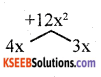Question 4.
Factorise the following
(i) 2x2 + 7x + 6
2x2 + 4x + 3x + 6
2x (x +2) + 3 (x + 2)
(x + 2) (2x + 3)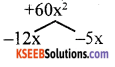(ii) 3x2 – 17x + 20
3x2 – 12x – 5x + 20
3x (x – 4) – 5 (x – 4)
(x – 4) (3x – 5)

(iii) 6x2 – 5x – 14
6x2 – 12x + 7x – 14
6x (x – 2) + 7 (x – 2)
(x – 2) (6x +7)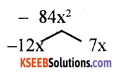(iv) 4x2 + 12xy – 5y2
4x2 – lOxy + 2xy + 5y2 – 20x2y2
2x (2x + 5y) + y
(2x + 5y)
(2x + 5y) (2x + y)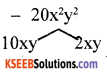(v) 4x4 – 5x2 + 1
4x2 – 4x2 – x2 + 1 4×4
4x2 (x2 – 1) – 1
(x2 – 1)
(x2 – 1) (4x2 – 1)
(x2 – 12) – ((2x)2 – 12)
(x2 – l2) – ((2x)2 – l2)
(x + 1) (x – 1) (2x + 1) (2x – 1)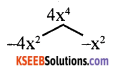Question 5.
Factorise the following
(i) x8 – y8
(x4)2 – (y4)2
= (x4 + y4) (x4 – y4)
= (x4 + y4) [(x2)2 – (y2)2]
= (x4 + y4) (x2 + y2) (x2 – y2)
= (x4 + y4) (x2 + y2) (x + y)
(x – y)

(ii) a12x4 – a4x12
[(a6)2(x2)2 – (a2)2(x6)2]
= [(a6x2)2 – (a2x6)2]
= (a6x2 + a2x6) (a6x2 – a2x6)
= a2x2 (a4 + x4)
[(a3)2 x2 – a2 (x3)2]
= a2x2 (a4 + x4) [(a3x)2 – (ax3)2] = a2x2 (a4 + x4) [(a3x + ax3)
(a3x – ax3)]
= a2x2 (a4 + x4) [ax(a2 + x2).ax (a2 – x2)]
= a2x2 (a4 + x4) [a2x2 (a2 + x2) (a2 – x2)]
= a4x4 (a4 + x4) (a2 + x2) (a + x) (a – x)

(iii) x4 + x2 + 1
X4 + X2 + 1 + X2 – X2
= x4 + 2x2 + 1 – x2
= (x2)2 + 2.x2.1 + l2 – x2
= (x2 + l)2 – X2
= (x2 + 1 + x) (x2 + 1 – x)
= (x2 + x + 1) (x2 – X + 1)

(iv) x4 + 5x2 + 9
x4 + 5x2 + 9 + x2 – x2
= x4 + 6x2 + 9 – x2
= (x2)2 + 2.x2.3 + 32 – x2 = (x2 + 3)2 – x2
= (x2 + 3 + x) (x2 + 3 – x)
= (x2 + x + 3) (x2 – x + 3)Question 6.
Factorise x4 + 4y4 use this to prove that 2011 4 + 64 is a composite number Answer:
x4 + 4y4
= (x2)2 + (2y2)2
= (x2 + 2y2)2 – 2x2.2y2
[v a2 + b2 = (a + b)2 – 2ab]
= (x2 + 2y2) – (4x2y2)
= (x2 + 2y2) – (2xy)2
(x2 + 2y2 + 2xy) (x2 + 2y2 – 2xy) [a2 – b2 = (a + b) (a – b)]
∴ x4 + 4y4 = (x2 + 2y2 + 2xy)
(x2 + 2y2 – 2xy)
Similarly 20114 + 64 can be written as
20114 + 4 x 16 = (2011)4 + 4.24
= (20112 + 2.22 + 2.2011.2) (20112 + 2.22 – 2.2011.2)
∴ 20114 + 64 is a composite number.error: Content is protected !!